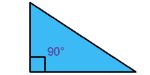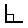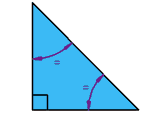# Right-Angled TrianglesA right-angled triangle (also called a right triangle) is a triangle with a right angle (90°) in it.The little square in the corner tells us it is a right angled triangle (I also put 90°, but you don't need to!)

The right angled triangle is one of the most useful shapes in all of mathematics!
(It is used in the Pythagoras Theorem and Sine, Cosine and Tangent for example).

Try it yourself (drag the points):

## Two Types

There are two types of right angled triangle:Isosceles right-angled triangle

One right angle
Two other equal angles always of 45°
Two equal sidesScalene right-angled triangle

One right angle
Two other unequal angles
No equal sides

### Example: The 3,4,5 TriangleThe "3,4,5 Triangle" has a right angle in it.

(Draw one if you ever need a right angle!)

It has no equal sides so it is a scalene right-angled triangle

And, like all triangles, the three angles always add up to 180°.